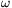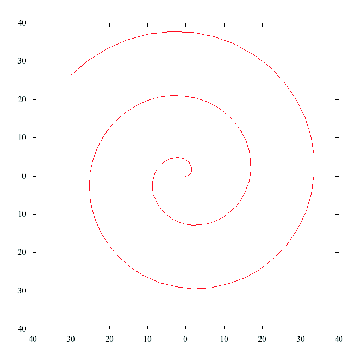Mathematical and Physical Journal
for High Schools
Issued by the MATFUND Foundation
 Already signed up? New to KöMaL?

#Problem P. 4329. (March 2011)

P. 4329. The jib of a tower crane is L=40 m long and it rotates about one of its ends in a horizontal plane at a constant angular speed of=0.03 s-1. On the jib a trolley moves away from the fixed end of the jib at a speed of u=0.08 m/s, with respect to the jib.

a) Determine the speed of the trolley with respect to the ground as a function of time.

b) Graph the path of the trolley with respect to the ground as it moves along the jib.

c) Determine the velocity of the trolley with respect to the ground as a function of time.

(4 pont)

Deadline expired on April 11, 2011.

Sorry, the solution is available only in Hungarian. Google translation

Megoldás. $\displaystyle a)$ A sebesség nagysága: $\displaystyle v=u\sqrt{1+\omega^2t^2}$.

$\displaystyle b)$$\displaystyle c)$ A sebességvektor

$\displaystyle v_x=u\left(\cos(\omega t)-\omega t\sin(\omega t)\right),$

$\displaystyle v_y=u\left((\sin(\omega t)+\omega t\cos(\omega t)\right).$

### Statistics:

 84 students sent a solution. 4 points: Agócs Fruzsina, Antalicz Balázs, Barta Szilveszter Marcell, Bedőházi Zsolt, Béres Bertold, Bolgár Dániel, Cseke Zsombor, Csóka József, Czigány Máté Gábor, Czipó Bence, Dávid Bence, Dinev Georgi, Filep Gábor, Fülep Andrea , Garami Anna, Horicsányi Attila, Iglói Gábor, Juhász Péter, Kaposvári Péter, Kovács 444 Áron, Laczkó Zoltán Balázs, Maknics András, Mázik László, Medek Ákos, Nagy Lajos, Nagy Zsolt, Nagy Zsuzsika, Papp Roland, Pázmán Koppány, Péterffy Gábor, Pető János, Sárvári Péter, Schwarcz Gergő, Szabó 928 Attila, Szélig Áron, Szemes Gábor Bence, Szentgyörgyi 994 Rita, Takács 737 Gábor, Tóth Balázs, Tuza Réka, Váncsa Szilárd, Varga 515 Balázs, Varga Zoltán Attila, Zahemszky Péter, Zsámboki Richárd. 3 points: Hoffmann Hanna, Jenei Márk, Nagyfi Péter, Pataki Bálint Ármin. 2 points: 27 students. 1 point: 6 students. 0 point: 1 student. Unfair, not evaluated: 1 solutions.

Problems in Physics of KöMaL, March 2011